# Chapter Four Utility Utility Functions u A utility

• Slides: 33Chapter Four Utility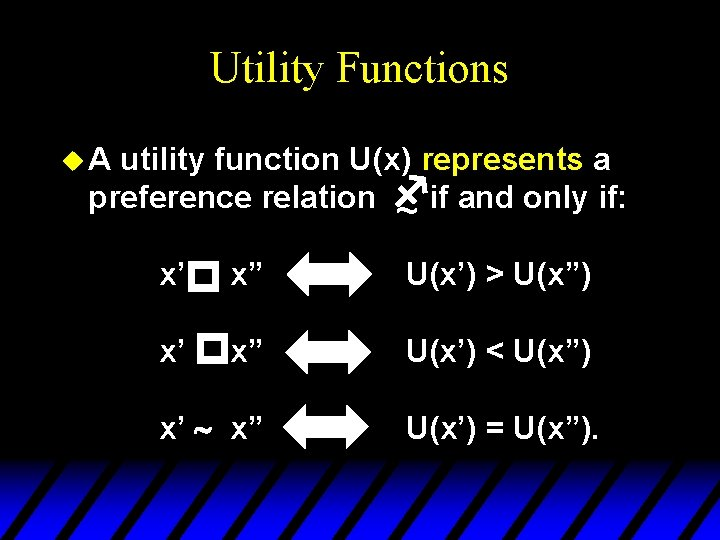Utility Functions u. A utility function U(x) represents a preference relation f ~ if and only if: p x’ x” U(x’) > U(x”) x’ px” U(x’) < U(x”) x’ ~ x” U(x’) = U(x”).Utility Functions u Utility is an ordinal concept; i. e. it is concerned only with order. u E. g. suppose U(x) = 6 and U(y) = 2. Then the bundle x is strictly preferred to the bundle y. It is not true that x is preferred three times as much as is y.Utility Functions & Indiff. Curves u Consider the bundles (4, 1), (2, 3) and (2, 2). u Suppose that (2, 3) (4, 1) ~ (2, 2). u Let us assign to these bundles any numbers that preserve this preference ordering; e. g. U(2, 3) = 6 and U(4, 1) = U(2, 2) = 4. u We will call these numbers utility levels. p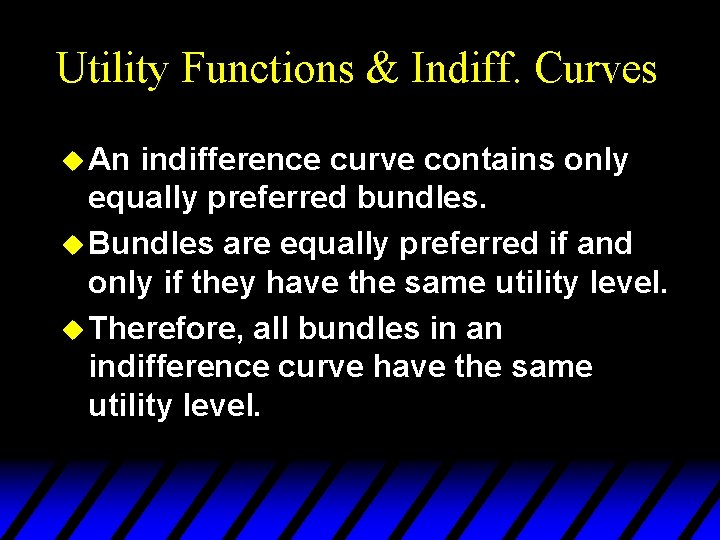Utility Functions & Indiff. Curves u An indifference curve contains only equally preferred bundles. u Bundles are equally preferred if and only if they have the same utility level. u Therefore, all bundles in an indifference curve have the same utility level.Utility Functions & Indiff. Curves u So the bundles (4, 1) and (2, 2) both lie in the indifference curve with the utility level U º 4 while the bundle (2, 3) lies in the indifference curve with the higher utility level U º 6. u On an indifference map diagram, this preference information looks as follows:Utility Functions & Indiff. Curves p x 2 (2, 3) (2, 2) ~ (4, 1) Uº 6 Uº 4 x 1Utility Functions & Indiff. Curves x 2 Uº 6 Uº 4 Uº 2 x 1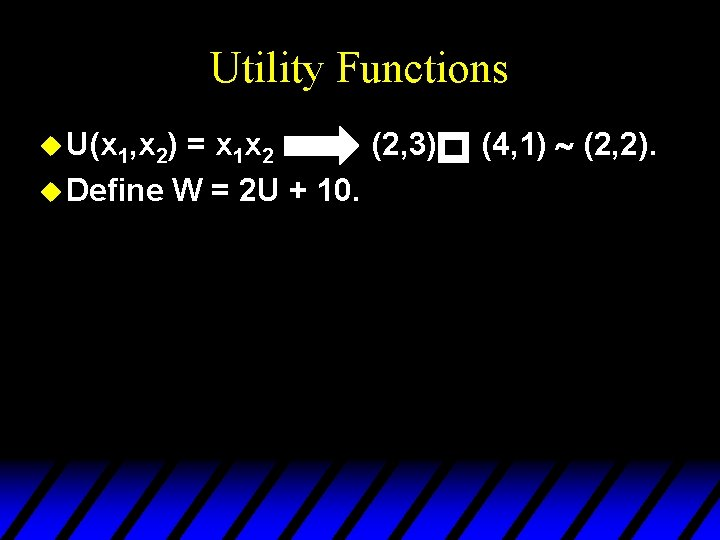Utility Functions p u U(x 1, x 2) = x 1 x 2 (2, 3) u Define W = 2 U + 10. (4, 1) ~ (2, 2).Utility Functions = x 1 x 2 (2, 3) (4, 1) ~ (2, 2). u Define W = 2 U + 10. u Then W(x 1, x 2) = 2 x 1 x 2+10 so W(2, 3) = 22 and W(4, 1) = W(2, 2) = 18. Again, (2, 3) (4, 1) ~ (2, 2). u W preserves the same order as U and V and so represents the same preferences. p u U(x 1, x 2) pGoods, Bads and Neutrals u. A good is a commodity unit which increases utility (gives a more preferred bundle). u A bad is a commodity unit which decreases utility (gives a less preferred bundle). u A neutral is a commodity unit which does not change utility (gives an equally preferred bundle).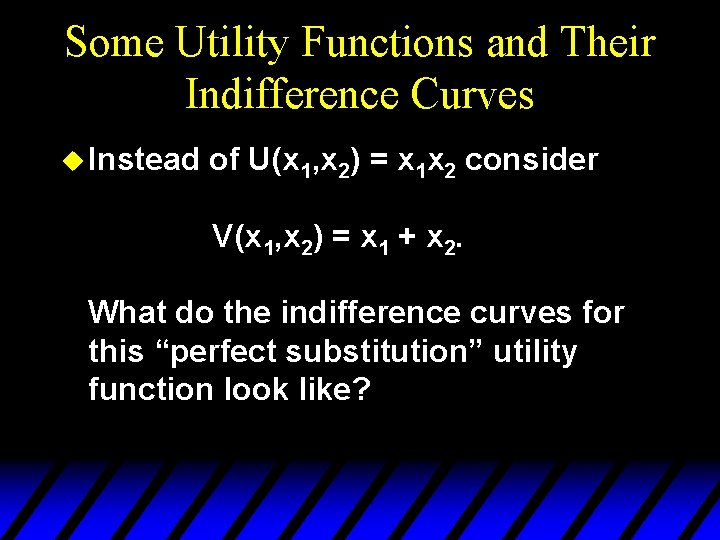Some Utility Functions and Their Indifference Curves u Instead of U(x 1, x 2) = x 1 x 2 consider V(x 1, x 2) = x 1 + x 2. What do the indifference curves for this “perfect substitution” utility function look like?Perfect Substitution Indifference Curves x 2 x 1 + x 2 = 5 13 x 1 + x 2 = 9 9 x 1 + x 2 = 13 5 V(x 1, x 2) = x 1 + x 2. 5 9 13 x 1 All are linear and parallel.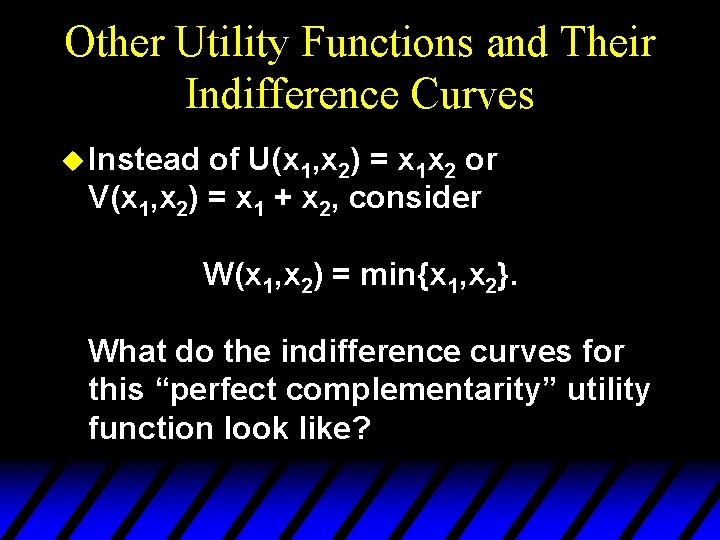Other Utility Functions and Their Indifference Curves u Instead of U(x 1, x 2) = x 1 x 2 or V(x 1, x 2) = x 1 + x 2, consider W(x 1, x 2) = min{x 1, x 2}. What do the indifference curves for this “perfect complementarity” utility function look like?x 2 8 5 3 Perfect Complementarity Indifference Curves 45 o W(x 1, x 2) = min{x 1, x 2} = 8 min{x 1, x 2} = 5 min{x 1, x 2} = 3 3 5 8 x 1 All are right-angled with vertices on a ray from the origin.Some Other Utility Functions and Their Indifference Curves u. A utility function of the form U(x 1, x 2) = f(x 1) + x 2 is linear in just x 2 and is called quasilinear. u E. g. U(x 1, x 2) = 2 x 11/2 + x 2.Quasi-linear Indifference Curves x 2 Each curve is a vertically shifted copy of the others. x 1Some Other Utility Functions and Their Indifference Curves u Any utility function of the form U(x 1, x 2) = x 1 a x 2 b with a > 0 and b > 0 is called a Cobb. Douglas utility function. u E. g. U(x 1, x 2) = x 11/2 x 21/2 (a = b = 1/2) V(x 1, x 2) = x 1 x 23 (a = 1, b = 3)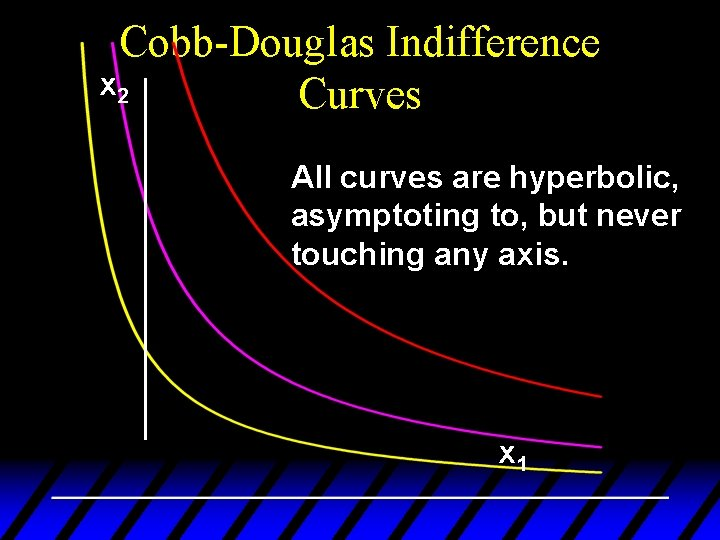Cobb-Douglas Indifference x 2 Curves All curves are hyperbolic, asymptoting to, but never touching any axis. x 1Marginal Utilities u Marginal means “incremental”. u The marginal utility of commodity i is the rate-of-change of total utility as the quantity of commodity i consumed changes; i. e.Marginal Utilities u E. g. if U(x 1, x 2) = x 11/2 x 22 thenMarginal Utilities u E. g. if U(x 1, x 2) = x 11/2 x 22 then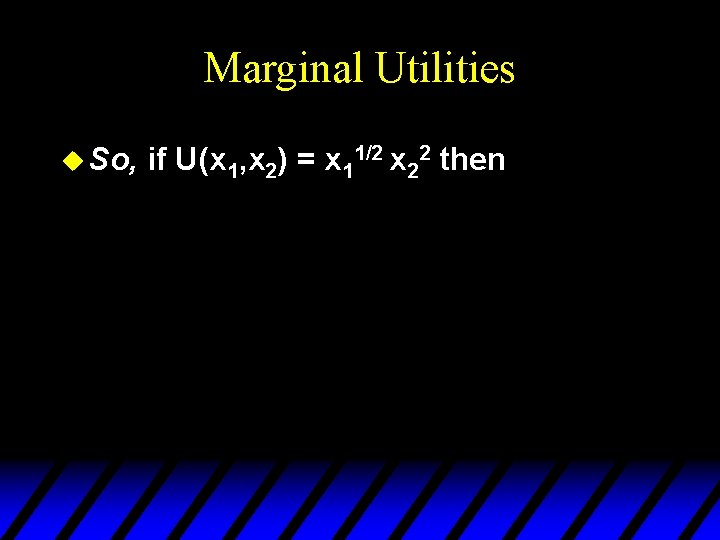Marginal Utilities u So, if U(x 1, x 2) = x 11/2 x 22 thenMarginal Utilities and Marginal Rates-of-Substitution u The general equation for an indifference curve is U(x 1, x 2) º k, a constant. Totally differentiating this identity gives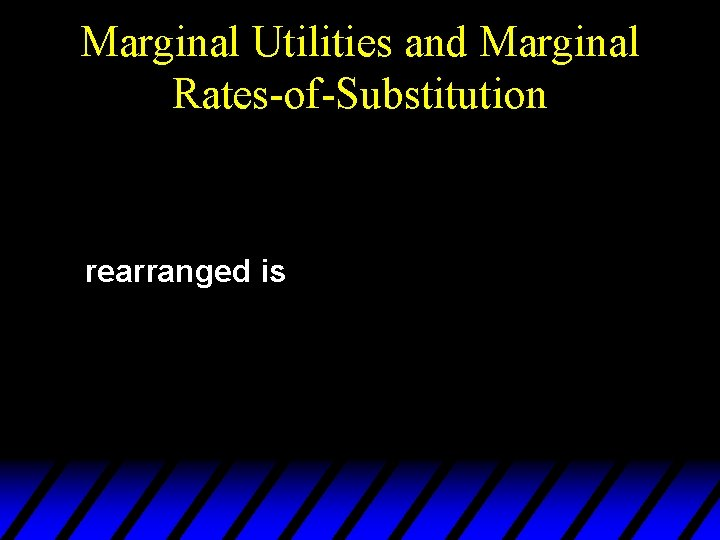Marginal Utilities and Marginal Rates-of-Substitution rearranged is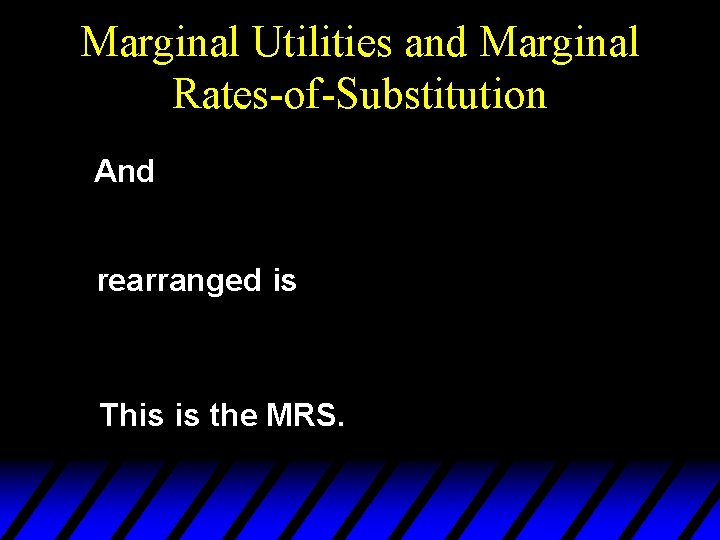Marginal Utilities and Marginal Rates-of-Substitution And rearranged is This is the MRS.Marg. Utilities & Marg. Rates-of. Substitution; An example u Suppose so U(x 1, x 2) = x 1 x 2. ThenMarg. Utilities & Marg. Rates-of. Substitution; An example U(x 1, x 2) = x 1 x 2; x 2 8 MRS(1, 8) = - 8/1 = -8 MRS(6, 6) = - 6/6 = -1. 6 U = 36 1 6 U=8 x 1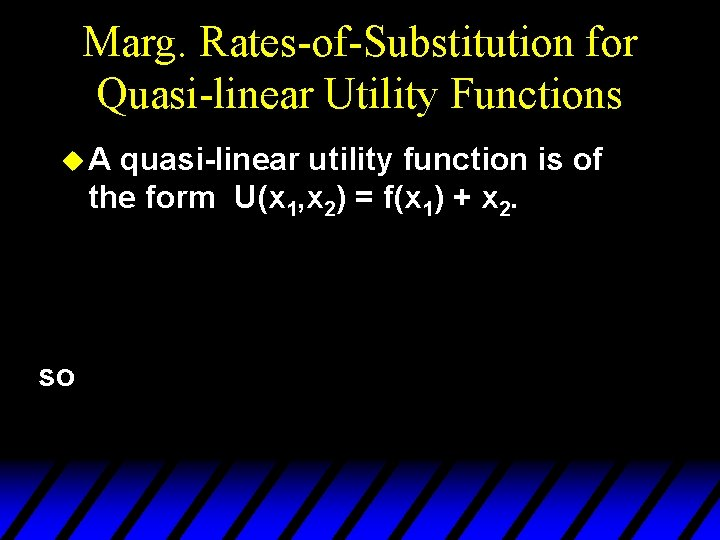Marg. Rates-of-Substitution for Quasi-linear Utility Functions u. A quasi-linear utility function is of the form U(x 1, x 2) = f(x 1) + x 2. so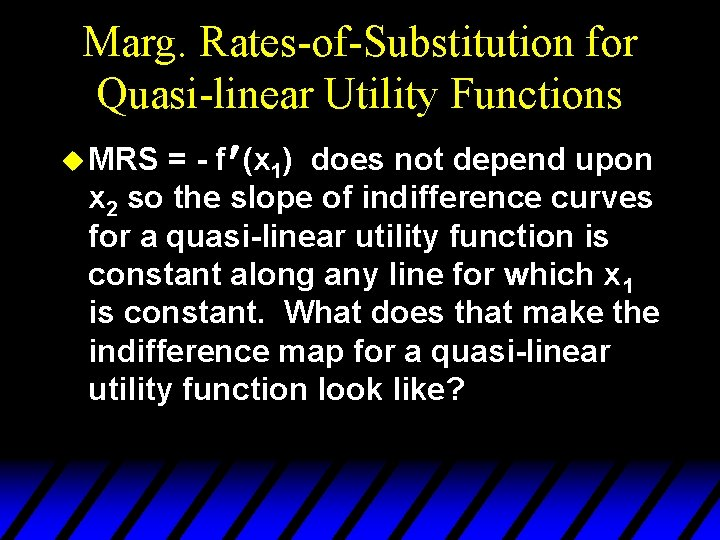Marg. Rates-of-Substitution for Quasi-linear Utility Functions = - f ¢ (x 1) does not depend upon x 2 so the slope of indifference curves for a quasi-linear utility function is constant along any line for which x 1 is constant. What does that make the indifference map for a quasi-linear utility function look like? u MRSx 2 Marg. Rates-of-Substitution for Quasi-linear Utility Functions MRS = - f(x 1’) Each curve is a vertically shifted copy of the others. MRS = -f(x 1”) MRS is a constant along any line for which x 1 is constant. x 1’ x 1” x 1Monotonic Transformations & Marginal Rates-of-Substitution u Applying a monotonic transformation to a utility function representing a preference relation simply creates another utility function representing the same preference relation. u What happens to marginal rates-ofsubstitution when a monotonic transformation is applied?Monotonic Transformations & Marginal Rates-of-Substitution u For U(x 1, x 2) = x 1 x 2 the MRS = - x 2/x 1. u Create V = U 2; i. e. V(x 1, x 2) = x 12 x 22. What is the MRS for V? which is the same as the MRS for U.